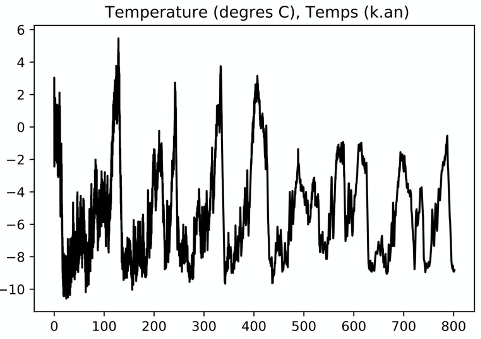## Data Science

3 ECTS

Contact: Frédéric CHAMBATAny set of data is a signal. In the sciences of the universe, data are sometimes provided in large amounts and processing them is a challenge in itself. But even in simple cases, it is useful to know the fundamentals: the notion of frequency, decomposition on a base of sinusoids, a Fourier spectrum etc.Temperature versus time (current = 0) recorded in the Dome C ice core. Signal processing allows, among other things, to identify periodicities in this type of curve.

Contents:

• Fourier II (18h)
• Dirac distribution (2h)
• Fourier transform, convolution, TF of usual functions, diff. eq. (6h)
• Signal processing (10h)
• Convolution and linear physics
• Green's functions
•  Truncation
•  Discretization
• DFT and FFT
• Apodisation, detrending
• Programming of these concepts (4 lab sessions)
• AI / Data science (6h)
• PCA (2h)
• Introduction to classification (k-means method) (2h)
• Introduction to neural networks (2h)